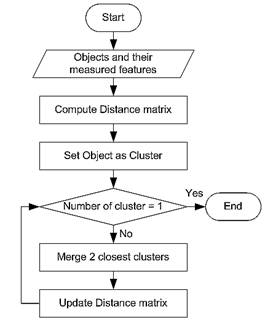## Hierarchical Clustering Algorithm

There are two main methods of hierarchical clustering algorithm.

First method is agglomerative approach , where we start from the bottom where all the objects are and going up ( bottom up approach ) through merging of objects. We begin with each individual objects and merge the two closest objects. The process is iterated until all objects are aggregated into a single group.

Second method is divisive approach (top down approach) , where we start with assumption that all objects are group into a single group and then we split the group into two recursively until each group consists of a single object. One possible way to perform divisive approach is to first form a minimum spanning tree (e.g using Kruskal algorithm) and then recursively (or iteratively) split the tree by the largest distance.

In this simple tutorial, I will only show the example of agglomerative approach.

Step by step algorithm of agglomerative approach to compute hierarchical clustering is as follow

1. Convert object features to distance matrix.
2. Set each object as a cluster (thus if we have 6 objects, we will have 6 clusters in the beginning)
3. Iterate until number of cluster is 1
1. Merge two closest clusters
2. Update distance matrix

The flow chart of agglomerative hierarchical clustering algorithm is given belowIn the next section , we will start with a simple numerical example.